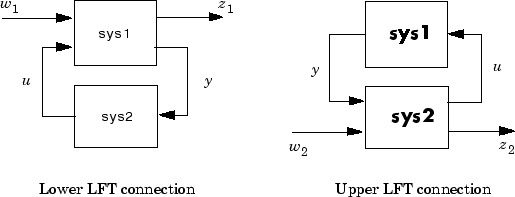Documentation

# lft

Generalized feedback interconnection of two models (Redheffer star product)

## Syntax

```lft sys = lft(sys1,sys2,nu,ny) ```

## Description

`lft ` forms the star product or linear fractional transformation (LFT) of two model objects or model arrays. Such interconnections are widely used in robust control techniques.

`sys = lft(sys1,sys2,nu,ny) ` forms the star product `sys` of the two models (or arrays) `sys1` and `sys2`. The star product amounts to the following feedback connection for single models (or for each model in an array).This feedback loop connects the first `nu` outputs of `sys2` to the last `nu` inputs of `sys1` (signals u), and the last `ny` outputs of `sys1` to the first `ny` inputs of `sys2` (signals y). The resulting system `sys` maps the input vector [w1 ; w2] to the output vector [z1 ; z2].

The abbreviated syntax

```sys = lft(sys1,sys2) ```

produces:

• The lower LFT of `sys1` and `sys2` if `sys2` has fewer inputs and outputs than `sys1`. This amounts to deleting w2 and z2 in the above diagram.

• The upper LFT of `sys1` and `sys2` if `sys1` has fewer inputs and outputs than `sys2`. This amounts to deleting w1 and z1 in the above diagram.## Limitations

There should be no algebraic loop in the feedback connection.

## Algorithms

The closed-loop model is derived by elementary state-space manipulations.